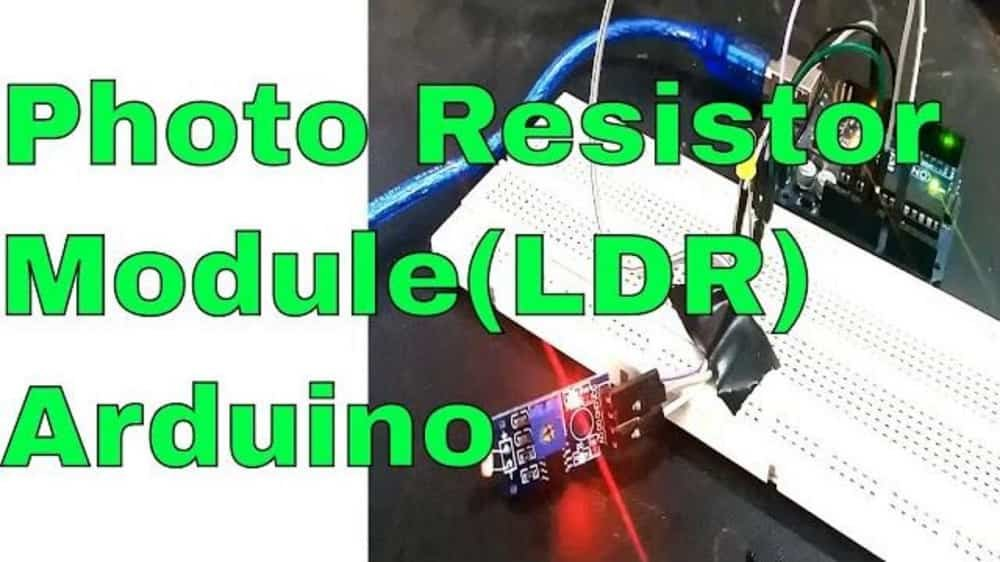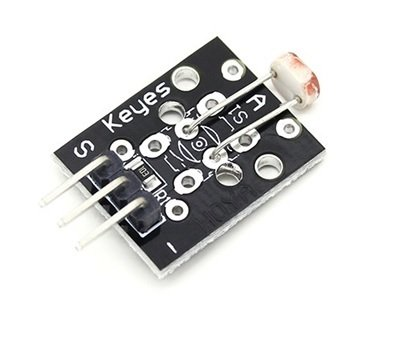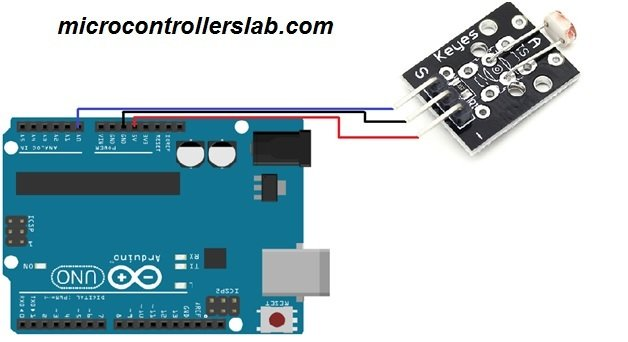# photo resistor interfacing with Arduino for light measurement

Photo Resistor Module: The photo resistor module is used to indicate the presence or absence of light or to measure that how much intensity of light is present. Photo resistor is also known as the light dependent resistor (LDR).The photo resistor module consists of a light dependent resistor (LDR). LDR is a simple and light sensitive resistor whose resistance changes with the amount of light falls on it. In dark, the resistance is very high, up to 1 M ohm, but when they are exposed to light then the resistivity decreases up to few ohms.Their sensitivity changes with the wavelength of light coming to it and they are non-linear devices. It has many applications in microcontrollers based projects. Some of them are given below:

When the intensity of light falling on the LDR will be higher, then its resistance will be lower and when the intensity of light falling on LDR will be lower, then its resistance will be higher. The module also has a 10K pull down resistor.This module is used in many projects where we need to automate the light. The applications of LDR are in cameras, street lights, detectors, light control devices.

### Specifications of Photo resistor module

• It operates on 5V DC.
• It is compatible with any microcontroller
• It is sensitive to temperature.

### Types of photo resistors

According to the features, the photo resistors are of three types; Ultraviolet photosensitive resistor, infrared light-sensitive resistor, Visible photosensitive resistor.

### Features of photo resistors

The main features of the photo resistors are as follows.

• Dark Current, Dark Resistance: When the light is not completely coming to the sensor then the flowing current is called the dark current. The ratio of the applied voltage and the dark current is known as the dark resistance.
• Sensitivity: The light depending resistor is light sensitive. When the light is coming to it then the resistance decreases and when it is exposed to light then the resistance increases.
• Volt-Ampere Characteristic Curve: The volt-Ampere characteristic curve of the LDR is such that when the light current with applied voltage increases. The volt-ampere characteristic curve is used to explain the voltage and current relationship.
• Temperature Coefficient: These are affected by temperature. When the temperature is low, the sensitivity will be high and at a high temperature the sensitivity will be low.
• Rated Power: When the temperature is higher, then the power consumed will be reduced.

### Pin Out of Photo Resistor Module

The photo resistor module has three pins. These pins from left to right are• S: Output pin
• +5v: Input voltage pin
• GND: Ground

### Photo resistor interfacing with Arduino

The connection scheme is very easier. You just have to connect three pins. Connect the 5V and the ground of the module with the 5V and the ground of the Arduino. Then connect the signal pin of module with the A0 of Arduino.

• Arduino A0 –> Module Signal (S)
• Arduino 5V –> Module +5V (Pin2)
• Arduino GND –> Module GND (-)

The Arduino will give us value between 0 to 1023. When there will be no light, then the value will be lower and when the intensity of light will be higher, then the value will be higher. We have set a threshold value in the code, which is 500. So, when the value will be lower than 500 then the Arduino will turn high the pin 13 where Arduino have a built in LED, which will glow up. You can also connect a led at any other pin you want.### Code of light measurement using LDR and Arduino

```// This code is for the Photo resistor Module.

int sensorPin = A0 ;                      // Selecting A0 as the sensor input pin.

int ledPin = 13 ;                          // Selecting pin 13 as the LED pin

int sensorValue = 0 ;                     // variable to store the value coming from the sensor

void setup ( ) {                          // code written in it will only run once.

pinMode ( ledPin , OUTPUT ) ;          // Declaring pin 13 as output pin

Serial.begin ( 9600 ) ;                // Setting baud rate at 9600

}

void loop ( )  {                         // Code written in it will run repeatedly

sensorValue = analogRead ( sensorPin ) ;          // Reading data from pin A0 and storing in Sensor value

if ( sensorValue < 500)

{

digitalWrite ( ledPin , HIGH ) ;                            // This will set the pin 13 as high

Serial.println ( " The value of LDR is = " ) ;              // This will print " The value of LDR is = " on display

Serial.println (sensorValue ) ;                             // This will print the value of LDR on display

delay ( 1000 ) ;                                            // This will give delay on 1 sec

}

else

{

digitalWrite ( ledPin , LOW ) ;                                    // This will set the pin 13 as low

Serial.println ( " The value of LDR is = " ) ;

Serial.println ( sensorValue ) ;                                    // This will print the sensor value on display

delay ( 1000 ) ;                                                                   // This will give delay of 1 sec

}

}```

This module has a wide range of applications. It can be used in many projects where you need to automate the light. You can also check electronics projects based on LDR for automatic street light control and auto intensity control of street lights.

You may also read: BH1750 Ambient Light Sensor Interfacing with Arduino